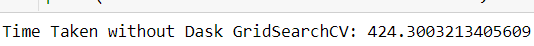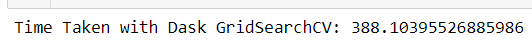Related Articles
DaskGridSearchCV – A competitor for GridSearchCV
• Last Updated : 06 Nov, 2020

The buzzwords in the field of Data Science such as Machine Learning, Artificial Intelligence and Deep Learning are appearing at the maximum number of places on the Internet in recent time. Everyone wants to try out different models of Machine Learning and Deep Learning and achieve the best results possible. There are some computational limits for some of the models. To get the best model in Machine Learning, there is something known as Hyperparameter Tuning.

Hyperparameter Tuning is basically getting the best set of parameters selected for a model. There are 2 common approaches to this: GridSearchCV and RandomizedSearchCV.
GridSearchCV is basically considering all the combinations of the candidates in finding the best parameters. This would in turn take a very long time when there are a greater number of parameter and their values to tune. There is an approach by which we can fasten this process. This is the main thing that occupies most of the time in Machine Learning. Before diving into the approach part let us skim through the basics of GridSearchCV and parallel computing concepts.

What is Grid Search?

GridSearchCV is a technique to search through the best parameter values from the given set of the grid of parameters. It is basically a cross-validation method. the model and the parameters are required to be fed in. Best parameter values are extracted and then the predictions are made.

Code: Python code explaining the working of GridSearchCV:

 `# Importing the libraries needed``pip install pandas``import` `pandas as pd``from` `sklearn.linear_model ``import` `LogisticRegression``from` `sklearn.svm ``import` `SVC``from` `sklearn.model_selection ``import` `GridSearchCV`` ` `# Loading the Dataset``# A standard dataset here is taken for better understanding.``iris ``=` `pd.read_csv(``'https://raw.githubusercontent.com/pranavkotak8/Datasets/master/Iris.csv'``)``target``=``iris[``'Species'``]``iris.drop(columns``=``{``'Id'``,``'Species'``},inplace``=``True``)`` ` `# Assigning the parameters and its values which need to be tuned.``parameters ``=` `{``'kernel'``: [``'linear'``, ``'rbf'``], ``'C'``:[``1``,``2``,``3``,``6``]}`` ` `# Fitting the SVM model``modelsvc ``=` `SVC()`` ` `# Performing the GridSearchCV``clf ``=` `GridSearchCV(modelsvc, parameters)``clf.fit(iris, target)`

Output:So, in the above code we saw how GridSearchCV can be implemented. In the above code, it was SVM model, similarly, other models can be used. The difference would be in parameters and their values would change. Here I have taken up 2 parameters so it’s faster, but what if we have more parameters or a complex model to fit? Let’s get straight to the answer for this.

Can we fasten up the process of GridSearchCV by any means?

So, the answer is yes, we can increase the speed of GridSearchCV. Okay, you must be wondering how. So, for that lets dive into how actually the GridSearchCV works.

Working of GridSearchCV:

GridSearchCV is a machine learning library for python. We have an exhaustive search over the specified parameter values for an estimator. An estimator object needs to provide basically a score function or any type of scoring must be passed. There are 2 main methods which can be implemented on GridSearchcv they are fit and predict. There are other also predict_proba,decision_function etc. But the two mentioned are frequently used. According to the type of algorithm which is been used for the dataset at hand for analysis it has its own different parameters. The user needs to give a different set of values for the important parameters. Gridsearchcv by cross-validations will find out the best value for the parameters mentioned. There are default values set for the parameters which can be also taken into consideration.

Intuition Behind GridSearchCV:

Every Data Scientist working on a model needs the best model for the final conclusive analysis. For this GridSearchCV can help build it. The program here is told to run a grid-search with cross-validations. The cross-validation followed in GridSearchCV is k-fold cross-validation approach. So basically in k-fold cross-validation, the given data is been spilt into k-folds depending on the need of the analyst where every fold at some of the other point of time is been used in testing. If for example K=3, then in the first iteration first fold is used to test the model and the rest folds are used to train the model. In the second iteration, the second fold is used to test the model and the first and the third fold is used to train the model. This is repeated unless every fold is used for testing. Evaluating like this the grid search takes into considerations all the combinations of parameters and finds the best possible model for the algorithm being used in the particular problem.

Below are the different methods listed with their use:

Methods:

Some of the Main Methods include as follows:

• fit() – This method takes the input data and fits all the hyperparameters values.
• predict(X) – Predictions are made on the given data X taking into consideration the best parameters found through the fit method.
• score() – It gives us the score after evaluating the data on the best parameters.
• get_params() – It gives us the list of the best parameters and their values.

You can download the data from the link

Code:

 `# Importing the libraries which are required:``import` `pandas as pd``import` `numpy as np``from` `sklearn.model_selection ``import` `train_test_split``from` `sklearn.preprocessing ``import` `MinMaxScaler``from` `sklearn.feature_selection ``import` `SelectKBest``from` `sklearn ``import` `svm``from` `sklearn.model_selection ``import` `RandomizedSearchCV,GridSearchCV``import` `warnings``warnings.filterwarnings(``'ignore'``)``import` `seaborn as sns``import` `matplotlib.pyplot as plt`` ` `# Reading the train data``train ``=` `pd.read_csv(``'C:\\Users\\prana\\Downloads\\smartphone_activity_dataset.csv'``)`` ` `# Dropping the target column``train.drop(columns``=``{``'activity'``},inplace``=``True``)`` ` `# Scaling the data``from` `sklearn.preprocessing ``import` `MinMaxScaler``t ``=` `MinMaxScaler()``train_f ``=` `t.fit_transform(train)``train_f ``=` `pd.DataFrame(train_f)`` ` `# Splitting into train and test set``X_train,X_test,y_train,y_test``=``train_test_split(train_f, ``            ``target, test_size ``=` `0.8``, random_state ``=` `100``)`` ` `# Importing the DaskGridSearchCV, importing time ``# and also running the gridsearchcv``# So here we are using DaskGridSearchCV. ``from` `dask_ml.model_selection ``import` `GridSearchCV as DaskGridSearchCV``start``=``time.time()`` ` `parameters``=``{``              ``'C'``: [``0.1``, ``1``,``5``, ``10``,``15``,``20``,``100``,``500``],  ``              ``'gamma'``: [``0.5``,``0.80``,``1``, ``0.1``], ``              ``'kernel'``: [``'rbf'``,``'linear'``,``'sigmoid'``]}``     ` `modelsvc``=``SVC()`` ` `gscv ``=` `DaskGridSearchCV(modelsvc, param_grid ``=` `parameters, cv ``=` `5``, n_jobs ``=` `-``1``)`` ` `grid_results ``=` `gscv.fit(X_train, y_train)``end ``=` `time.time()``print``(``"Time Taken with Dask GridSearchCV:"``, end``-``start)`` ` `# Importing the GridSearchCV, importing time and ``# also running the gridsearchcv``# So here we are using the normal GridSearchCV method to implement``# the same algorithm and same parameters with the same set of values. ``# This is merely done to compare and measure the computational time for both the methods.``start ``=` `time.time()``gscv ``=` `GridSearchCV(svm.SVC(),  {``              ``'C'``: [``0.1``, ``1``,``5``, ``10``,``15``,``20``,``100``,``500``],  ``              ``'gamma'``: [``0.5``,``0.80``,``1``, ``0.1``], ``              ``'kernel'``: [``'rbf'``,``'linear'``,``'sigmoid'``]``},cv ``=` `5``,return_train_score ``=` `False``,n_jobs ``=` `-``1``)`` ` `grid_results ``=` `gscv.fit(X_train, y_train)``end ``=` `time.time()``print``(``"Time Taken without Dask GridSearchCV:"``, end``-``start)`

Output:Comparison between the Scikit-learn version and the Dask-version of GridSearchCV:

Conclusion:

As it is evidently seen from the output, we can say that DaskGridSearchCV is 1.09 times faster than normal GridSearchCV. We have in turn reduced the time for searching for the best parameter values. This can be applied to other algorithms and also more set of parameters also.

Following are some key points to take into consideration while applying Dask-SearchCV:

• If the model has pipeline and the early steps are costly then you would inherit performance benefits.
• The Data which you are trying to fit is already on a cluster, then Dask-SearchCV will perform really better since it works well on remote data.
• If your data is very large then this will not help much. It is meant for scheduling Scikit-Learn estimator fits on a small to medium scale data.

References: sklearn.model_selection.GridSearchCV.htmlMy Personal Notes arrow_drop_up